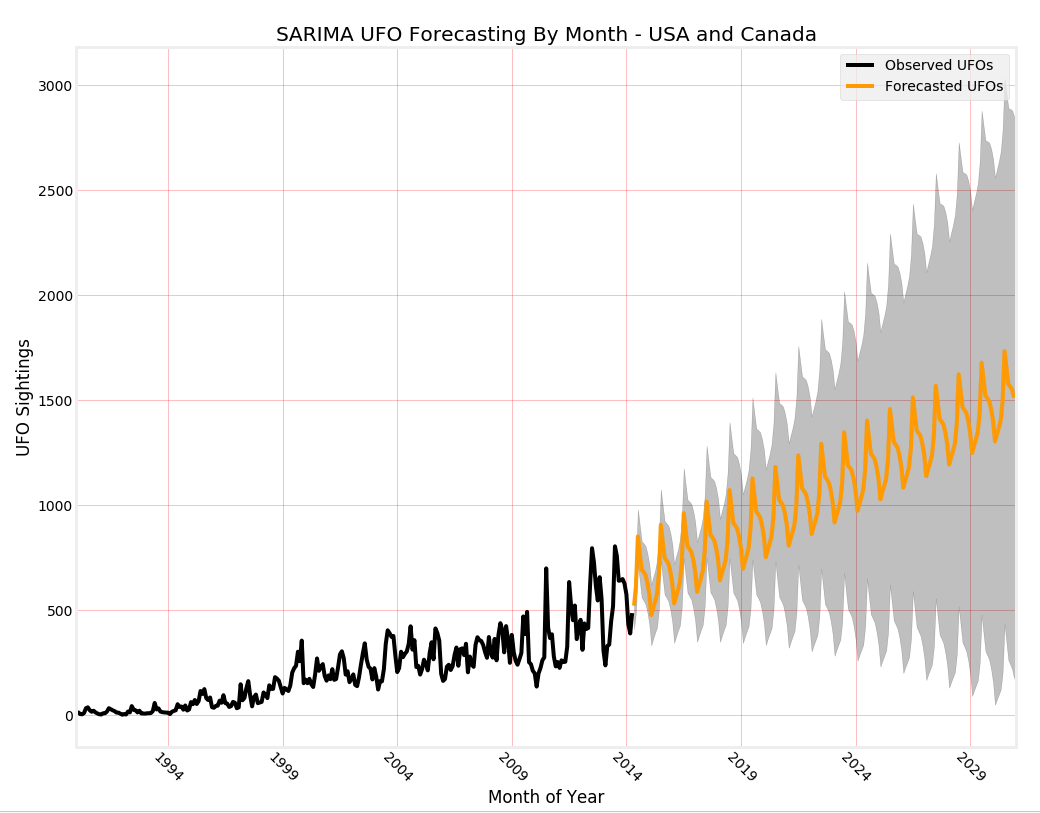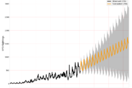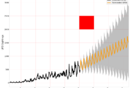# [Halloween Data Visualization Competition] Forecasting UFO Sightings With SARIMA ModellingAfter some exploration of the UFO data, I suspected there might be a seasonal component. Plotting UFO sightings by month reveals somewhat regular peaks and valleys.

Seemed like a great dataset to try SARIMA (Seasonal Auto Regressive Integrated Moving Average) forecasting (H/T to Data Scientist Susan Li)

My visualization plots the observed UFOs (black line) by month up until April 2014, and then uses the predictions generated by the model to forecast all the way to 2030 (orange line). The forecast reveals the seasonality lurking within the data!

The shaded dark grey area represents the confidence interval of the forecast, which expands the further out we try to forecast.

I used black, orange, and red plotting colors to represent the halloween spirit. I also thought it was quite fitting that the forecast shading looks like the mouth of a certain venomous extraterrestrial who just had a huge box office opening this month 😏. I shaded in the red square to make it look more like an actual monster!

Code is below. Thanks for reading, this contest was a really fun idea!

```### SQL Query To Pull Data ###
select
left(date_observed, 7) as month_observed
, count(*) as "sum"
from
[kaggle_ufo_data]
where
country != ' '
and state != ' '
and country in ('us', 'ca')
and date_observed between '1990-01-01' and '2014-04-30'
group by
1
order by
1

### Python Script ###
import pandas as pd
import matplotlib.pyplot as plt
import statsmodels.api as sm
import itertools
plt.style.use('fivethirtyeight')

# Set datetime index and y variable
df['month_observed'] = pd.to_datetime(df['month_observed'])
df.set_index(df['month_observed'], inplace=True)
y = df['sum']

# Instantiate SARIMA model and fit to data
mod = sm.tsa.statespace.SARIMAX(y, order=(1,1,1), seasonal_order=(0,1,1,12), enforce_stationarity=False, enforce_invertibility=False)
results = mod.fit()

# Forecast 200 months ahead of April 2014
pred_uc = results.get_forecast(steps=200)
pred_ci = pred_uc.conf_int()

# Create the plot
ax = y.plot(label='Observed UFOs', figsize=(15,12), color='black')
pred_uc.predicted_mean.plot(ax=ax, label='Forecasted UFOs', color='orange')

# Format the plot
ax.fill_between(pred_ci.index, pred_ci.iloc[:,0], pred_ci.iloc[:,1], color='k', alpha=.25)
ax.fill_between(['2014-01','2019-01'], 2000,2500, color='r')
ax.set_xlabel('Month of Year')
ax.set_ylabel('UFO Sightings')
ax.tick_params(grid_color='r', grid_alpha=0.25)
ax.set_xlim(['1990-01','2031-01'])
plt.legend()
plt.xticks(rotation=-45)
plt.title('SARIMA UFO Forecasting By Month - USA and Canada')

# Output to Periscope
periscope.output(ax)
```